# Spherical mirrorsPage 1

#### WATCH ALL SLIDES

Slide 1SPHERICAL MIRRORS

Slide 2## What is a spherical mirror?

Spherical mirrors are mirrors whose surfaces form a part of a hollow sphere, The spheres can be made of glass or any polished metal surfaces

Types of spherical mirrors :

1. Convex Mirror

2. Concave Mirror

Slide 3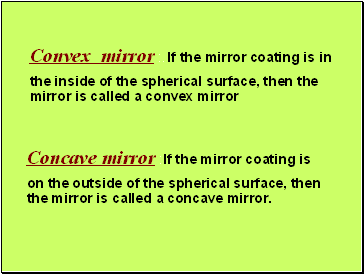Convex mirror :. If the mirror coating is in the inside of the spherical surface, then the mirror is called a convex mirror

Concave mirror :If the mirror coating is on the outside of the spherical surface, then the mirror is called a concave mirror.

Slide 4Slide 5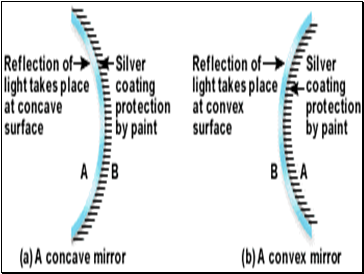Slide 6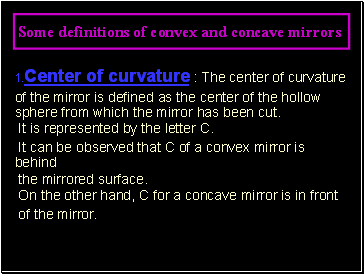## Some definitions of convex and concave mirrors

1.Center of curvature : The center of curvature of the mirror is defined as the center of the hollow sphere from which the mirror has been cut.

It is represented by the letter C.

It can be observed that C of a convex mirror is behind

the mirrored surface.

On the other hand, C for a concave mirror is in front

of the mirror.

Slide 7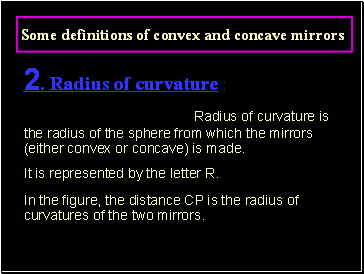Some definitions of convex and concave mirrors

Radius of curvature is the radius of the sphere from which the mirrors (either convex or concave) is made.

It is represented by the letter R.

In the figure, the distance CP is the radius of curvatures of the two mirrors.

Slide 8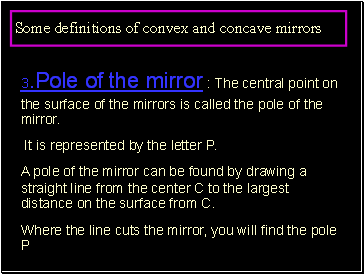Some definitions of convex and concave mirrors

3.Pole of the mirror : The central point on the surface of the mirrors is called the pole of the mirror.

It is represented by the letter P.

A pole of the mirror can be found by drawing a straight line from the center C to the largest distance on the surface from C.

Where the line cuts the mirror, you will find the pole P

Slide 9Some definitions of convex and concave mirrors

4.Principal axis of the mirror :

A straight line that passes through the center of curvature C and the pole P of the mirror, is called the principal axis of the mirror.

In the figure line XY is the principal axis of the mirrors.

The principal axis of a spherical mirror, functions similar to the normal of the plane mirror.

A ray of light passing along the principal axis will be reflected back along the same path.

Go to page:
1  2